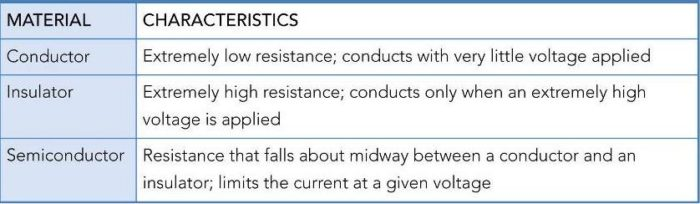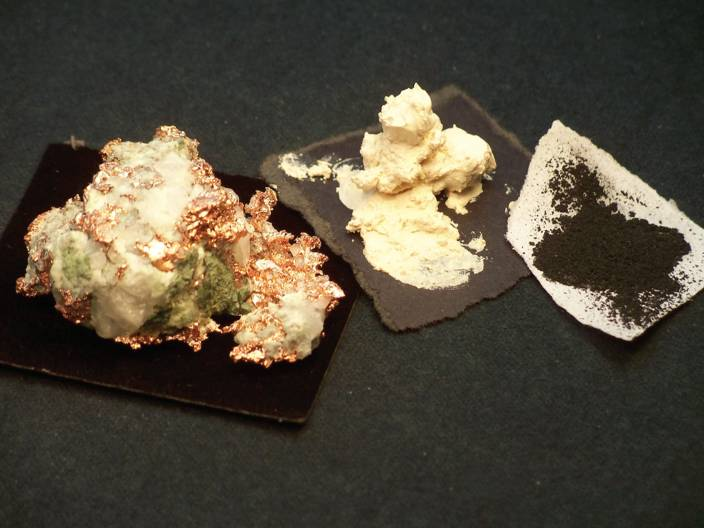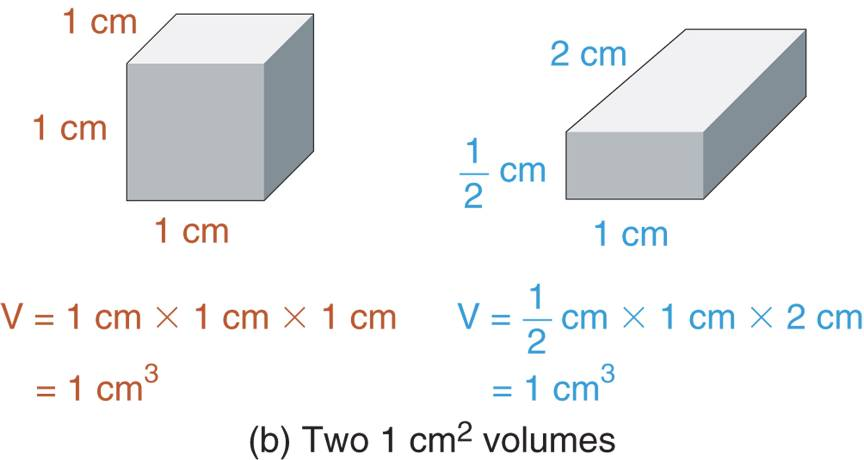Home / Basic Electrical / Conductors, Insulators, and Semiconductors | Theory | Examples

# Conductors, Insulators, and Semiconductors | Theory | Examples

Want create site? Find Free WordPress Themes and plugins.

All materials are classified according to their ability (or inability) to conduct as shown in Table 1.

Table 1 Conductor, Insulator, and Semiconductor CharacteristicsA good example of a Conductor is copper. Copper wire (which is the most commonly used conductor) passes current with little opposition.

A good example of an Insulator is a rubber. Rubber is used to coat the handles of many tools that are used in electric work (such as pliers, screwdrivers, etc.). It takes an extremely high voltage to force rubber to conduct.

A good example of a semiconductor is a graphite (a form of carbon), which is used to make many resistors. Graphite limits the amount of current that can be generated by the given amount of voltage.

In this article, we take a look at some of the characteristics of conductors, insulators, and semiconductors.

Conductors

A conductor is a material that provides little opposition to the flow of electrons (current).

Because the resistance of a conductor is low, very little energy is required to generate current through it. Thus, the materials with the lowest resistance make the best conductors. The valence shell of an atom determines its electrical characteristics. The conductivity of an element is determined by:

1. The number of valence shells electrons. The fewer valence shell electrons that an atom contains, the easier it is to force the atom to give up free electrons. The best conductors contain one valence electron per atom.
2. The number of atoms per unit volume. With more atoms per unit volume, a given voltage can generate more free electrons. The best conductors contain a high number of atoms per unit volume.Figure 1 Copper, rubber, and graphite.

Insulators

Insulators are materials that have extremely high resistance, thus block the flow of current.

For example, the insulation that covers a power cord prevents the current in the cord from reaching you when it is touched. Some elements (like neon) are natural insulators. Most of the insulators used to protect technicians in the field are compounds like rubber, Teflon, and mica (among others).

As you have probably guessed, conductors and insulators have opposite characteristics. In general, insulators are materials that have:

1. Complete valence shell (Eight valence electrons).
2. Relatively few atoms per unit volume.

With this combination of characteristics, an extremely high voltage is required to force an insulator into conduction.Figure 2 Some common insulated tools

Semiconductor

Any element or material with resistance that falls midway between that of a conductor and that of an insulator.

Semiconductors are materials that are neither good conductors nor good insulators. For example, graphite (a form of carbon) does not conduct well enough to be considered a conductor. At the same time, it does not block current well enough to be considered an insulator. Some other examples of semiconductors are silicon, germanium, and gallium arsenide.

In general, semiconductors have the following characteristics:

1. Valence shells that are half complete (i.e., contain four valence electrons)
2. A relatively high number of atoms per unit volume.

## Conductor Resistance

The resistance of the conductor (at a specified temperature) depends on:

1. The resistivity of the conductor.
2. The length of the conductor.
3. The cross-sectional area of the conductor.

Resistivity is the resistance of a specific volume of an element or compound.

The lower the resistivity of a conductor; the better it conducts. For example, the resistivity of copper is lower than the resistivity of aluminum. In fact, a 1000ft length of copper wire has just over half resistance of a comparable length of aluminum wire.

Resistivity is commonly rated using one of two units of measure:

1. CIRCULAR – MIL – OHMS PER FOOT (CML-Ω/FT)
2. OHM-CENTIMETERS (Ω-CM)

The volumes used in these two ratings are illustrated in Figure 3. Note that the NEC ® deals exclusively with circular mills.Figure 3 Volumes used to rate the resistivity of a conductor.

The mil equals one thousandth of an inch (0.001 in). The Circular Mil (CMIL) is a measure of area found by squaring the diameter (in mils) of the conductor.

One CIRCULAR-MIL FOOT (CMIL-FT) of material has a diameter of one mil and a length of one foot as shown in Figure 3a. When this volume of material is used to measure the resistivity of a given material, the rating is given in circular-mil-ohms per foot (cmil-Ω/ft).

The second unit of volume used for rating resistivity is the cubic centimeter. When this volume is used to measure the resistivity of a given material, the rating is given in ohm-centimeters (Ω-cm).

Table 2 shows the resistivity ratings of some elements that are commonly found in electrical and electronic circuits.

Table 2 Resistivity Ratings of Some Common Elements## Calculating the Resistance of a Conductor

When the length and cross-sectional area of a conductor are known, the resistance of the conductor can be calculated using:

$\begin{matrix}R=\rho \frac{L}{A} & {} & \left( 1 \right) \\\end{matrix}$

Where

ρ= the resistivity of the material

l= the length of the conductor

A= the cross-sectional area of the conductor

The Greek letter rho ρ in equation 1 is commonly used to represent resistivity. The following examples illustrate the calculation of the resistance of a conductor.

Example 1

Calculate the resistance of a 1000 ft length of copper that has a diameter of 81 mils.

Solution

According to table 2, copper has a resistivity rating of 10.37 cmil-Ω/ft. With a diameter of 81 mils, the cross-sectional area is found as:

$Area={{\left( \text{81 mils} \right)}^{2}}=6561\text{ cmils}$

Now, using equation 1, the resistance of the copper is found as:

$R=\rho \frac{L}{A}=\left( 10.37\frac{cmil-\Omega }{ft} \right)\times \frac{1000\text{ }ft}{6561\text{ }cmils}=1.58\Omega$

With this amount of resistance, it is easy to see that copper provides very little opposition to current.

What Equation 1 Shows Us?

Every equation serves two purposes. First, it shows us how to calculate the value of interest. Second (and probably most important), it shows us the relationship between the value of interest and its variables. For example, Equation 1 states that,

$\begin{matrix}R=\rho \frac{L}{A} & {} & \left( 1 \right) \\\end{matrix}$

The relationship shows us that:

1. The resistance of a conductor is directly proportional to its resistivity. That is, increases (or decreases) in resistivity cause a similar change in resistance.
2. The resistance of a conductor is directly proportional to its length. That is, the longer the conductor, the higher its resistance.
3. The resistance of the conductor is inversely proportional to its cross-sectional area. Thus, the greater the diameter of the conductor, the lower its resistance.

## Temperature and Resistance

The calculation in example assumes an operating temperature of 20oC. The resistivity of a conductor is affected by operating temperature. The effect of temperature on the resistivity of a material depends on its temperature coefficient.

A Positive Temperature Coefficient is a rating that indicates that the resistance of a given material increases when temperature increases. A Negative Temperature Coefficient is a rating which indicates that the resistance of a given material decreases when temperature increases and vice versa.

Conductors have positive temperature coefficients. Thus, the resistance of a conductor increases when temperature increases.

Review Questions

What is conductor? An insulator? A semiconductor?

A conductor has low resistance and has 1 valence electron. An insulator has high resistance and has 8 valence electrons. A semiconductor falls midway between an insulator and a conductor. Semiconductors have 4 valence electrons.

List the factors that determine the conductivity of an element?

Conductivity is determined by the number of valence electrons and the number of atoms per unit volume.

What is resistivity?

Resistivity is the resistance of a specific volume of a material.

Describe the units of measure that are commonly used to express resistivity?

Resistivity is defined in circular-mil-ohms per foot (CMIL-Ω/ft), or in ohm-centimeters (Ω-cm).

What is s positive temperature coefficient?

A positive temperature coefficient defines how much the resistance of a material increases with temperature.

What is s negative temperature coefficient?

A negative temperature coefficient defines how much the resistance of a material decreases with temperature.

What is the temperature coefficient for a conductor?

Conductors have a positive temperature coefficient.

Did you find apk for android? You can find new Free Android Games and apps.